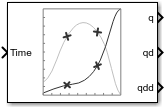# Polynomial Trajectory

Generate polynomial trajectories through waypoints

• Library:
• Robotics System Toolbox / Utilities

•## Description

The Polynomial Trajectory block generates trajectories to travel through waypoints at the given time points using either cubic, quintic, or B-spline polynomials. The block outputs positions, velocities, and accelerations for achieving this trajectory based on the Time input. For B-spline polynomials, the waypoints actually define the control points for the convex hull of the B-spline instead of the actual waypoints, but the first and last waypoint are still met.

The initial and final values are held constant outside the time period defined in Time points.

## Ports

### Input

expand all

Time point along the trajectory, specified as a scalar or vector. In general, when specified as a scalar, this value is synced with simulation time and is used to specify the time point for sampling the trajectory. The block outputs a vector of the trajectory variables at that instant in time. If the time is specified as a vector, the block outputs a matrix with each column corresponding to each element of the vector.

Data Types: `single` | `double`

Positions of waypoints of the trajectory at given time points, specified as an n-by-p matrix, where n is the dimension of the trajectory and p is the number of waypoints. If you specify the Method as `B-spline`, these waypoints actually define the control points for the convex hull of the B-spline, but the first and last waypoint are still met.

#### Dependencies

To enable this input, set Waypoint Source to `External`.

Time points for waypoints of trajectory, specified as a p-element vector.

#### Dependencies

To enable this input, set Waypoint Source to `External`.

Velocity boundary conditions for waypoints, specified as an n-by-p matrix. Each row corresponds to the velocity at each of the p waypoints for the respective variable in the trajectory.

#### Dependencies

To enable this input, set Method to ```Cubic Polynomial``` or `Quintic Polynomial` and Parameter Source to `External`.

Acceleration boundary conditions for waypoints, specified as an n-by-p matrix. Each row corresponds to the acceleration at each of the p waypoints for the respective variable in the trajectory.

#### Dependencies

To enable this parameter, set Method to ```Quintic Polynomial``` and Parameter Source to `External`.

### Output

expand all

Position of the trajectory, specified as a scalar, vector, or matrix. If you specify a scalar for the Time input with an n-dimensional trajectory, the output is a vector with n elements. If you specify a vector of m elements for the Time input, the output is an n-by-m matrix.

Data Types: `single` | `double`

Velocity of the trajectory, specified as a scalar, vector, or matrix. If you specify a scalar for the Time input with an n-dimensional trajectory, the output is a vector with n elements. If you specify a vector of m elements for the Time input, the output is an n-by-m matrix.

Data Types: `single` | `double`

Acceleration of the trajectory, specified as a scalar, vector, or matrix. If you specify a scalar for the Time input with an n-dimensional trajectory, the output is a vector with n elements. If you specify a vector of m elements for the Time input, the output is an n-by-m matrix.

Data Types: `single` | `double`

## Parameters

expand all

Specify `External` to specify the Waypoints and Time points parameters as block inputs instead of block parameters.

Positions of waypoints of the trajectory at given time points, specified as an n-by-p matrix, where n is the dimension of the trajectory and p is the number of waypoints. If you specify the Method as `B-spline`, these waypoints actually define the control points for the convex hull of the B-spline, but the first and last waypoint are still met.

#### Dependencies

To specify this parameter in the block mask, set Waypoint Source to `Internal`.

Time points for waypoints of trajectory, specified as a p-element vector, where p is the number of waypoints.

#### Dependencies

To specify this parameter in the block mask, set Waypoint Source to `Internal`.

Method for trajectory generation, specified as either ```Cubic Polynomial```, `Quintic Polynomial`, or `B-Spline`.

Specify `External` to specify the Velocity boundary conditions and Acceleration boundary conditions parameters as block inputs instead of block parameters.

Velocity boundary conditions for waypoints, specified as an n-by-p matrix. Each row corresponds to the velocity at each of the p waypoints for the respective variable in the trajectory.

#### Dependencies

To enable this input, set Method to ```Cubic Polynomial``` or `Quintic Polynomial`.

Acceleration boundary conditions for waypoints, specified as an n-by-p matrix. Each row corresponds to the acceleration at each of the p waypoints for the respective variable in the trajectory.

#### Dependencies

To enable this parameter, set Method to ```Quintic Polynomial```.

• `Interpreted execution` — Simulate model using the MATLAB® interpreter. This option shortens startup time but has a slower simulation speed than `Code generation`. In this mode, you can debug the source code of the block.

• `Code generation` — Simulate model using generated C code. The first time you run a simulation, Simulink® generates C code for the block. The C code is reused for subsequent simulations, as long as the model does not change. This option requires additional startup time, but the speed of the subsequent simulations is comparable to `Interpreted execution`.

 Farin, Gerald E. Curves and Surfaces for Computer Aided Geometric Design: A Practical Guide. San Diego, CA: Academic Press, 1993.